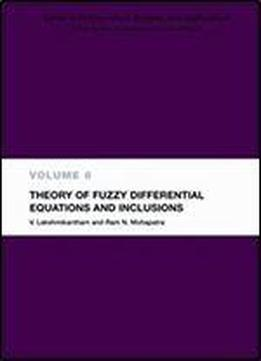# Theory Of Fuzzy Differential Equations And Inclusions (mathematical Analysis And Applications) by V. Lakshmikantham / 2003 / English / DjVu

Fuzzy differential functions are applicable to real-world problems in engineering, computer science, and social science. That relevance makes for rapid development of new ideas and theories. This volume is a timely introduction to the subject that describes the current state of the theory of fuzzy differential equations and inclusions and provides a systematic account of recent developments. The chapters are presented in a clear and logical way and include the preliminary material for fuzzy set theory a description of calculus for fuzzy functions, an investigation of the basic theory of fuzzy differential equations, and an introduction to fuzzy differential inclusionsFuzzy differential functions are applicable to real-world problems in engineering, computer science, and social science. That relevance makes for rapid development of new ideas and theories. This volume is a timely introduction to the subject that describes the current state of the theory of fuzzy differential equations and inclusions and provides a systematic account of recent developments. The chapters are presented in a clear and logical way and include the preliminary material for fuzzy set theory a description of calculus for fuzzy functions, an investigation of the basic theory of fuzzy differential equations, and an introduction to fuzzy differential inclusions.

views: 573